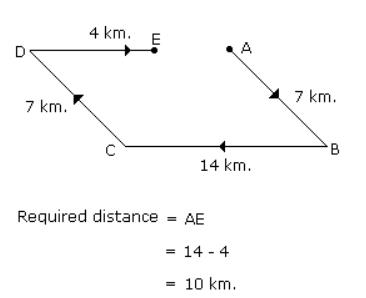Courses

# Test: Rest And Motion

## 10 Questions MCQ Test Science Class 9 | Test: Rest And Motion

Description
This mock test of Test: Rest And Motion for Class 9 helps you for every Class 9 entrance exam. This contains 10 Multiple Choice Questions for Class 9 Test: Rest And Motion (mcq) to study with solutions a complete question bank. The solved questions answers in this Test: Rest And Motion quiz give you a good mix of easy questions and tough questions. Class 9 students definitely take this Test: Rest And Motion exercise for a better result in the exam. You can find other Test: Rest And Motion extra questions, long questions & short questions for Class 9 on EduRev as well by searching above.
QUESTION: 1

### Rest state and motion of body are

Solution:

Rest and motion are the relative terms because they depend on the observer’s frame of reference.
If an observer is at rest in his or her own frame of reference, but he may be moving in other observer's frame of reference. So if two different observers are not at rest with respect to each other, then they too get different results when they observe the motion or rest of a body.

QUESTION: 2

### An ant moves from one corner of a hall to the diagonally opposite corner. If the dimension of the hall are 8 m x 6 m, the displacement of the ant is :

Solution:

In this case
Displacement = hypotenuse
Displacement = (82 +62)1/2 = 10 m

QUESTION: 3

### The displacement covered by a seconds’ hand of radius r in a clock after one revolution is:

Solution:

"Displacement will be zero because displacement is the difference between initial and final position but after one revolution the second hand came to its initial position; so it's final and initial position is same so displacement is zero "

QUESTION: 4

A man leaves his house at 7:30 a.m. for a morning walk, and returns back at 8:15 a.m. after covering 3 km. Is displacement in this time is:

Solution:

His displacement will be zero although distance covered is non-zero since he ends up where he started.

Displacement can be zero but distance cannot be zero because distance is the total path covered but displacement is the shortest distance between the initial and final position. It can be zero at the end of one round.

QUESTION: 5

A body travels from A to B at 40m/s and from B to A at 60m/s. Calculate the average speed.

Solution:

Total time taken by the body to travel from A to B and then from B to A,
t1+t2 = AB/40 + AB/60 = AB/24 s
Total distance covered = AB + BA = 2AB
Average speed = 2AB/(t1+t2) = 48m/s

QUESTION: 6

A car moved 60 km East and 90 km West. What is the distance?

Solution:

60km.east + 90km.west =150km.

QUESTION: 7

A body is said to be in non-uniform motion if it travels

Solution:

Non Uniform motion is defined as the motion of an object in which the object travels with varied speed and it does not cover same distance in equal time intervals, irrespective of the time interval length.

Examples:

1. If a car covers 10 meters in first two seconds, and 15 meters in next two seconds.

2. The motion of a train.

QUESTION: 8

A Quantity which has both magnitude and direction is called.

Solution:

Vector quantity has both magnitude and direction but scalar quantity have only magnitude

QUESTION: 9

If a body does not changes its position with respect to its surroundings, it is said to be at

Solution:

A body whose position with respect to surrounding doesn't change is said to be in a state of rest .

QUESTION: 10

Radha moves towards South-East a distance of 7 km, then she moves towards West and travels a distance of 14 km. From here she moves towards North-West a distance of 7 km and finally she moves a distance of 4 km towards east. How far is she now from the starting point?

Solution: